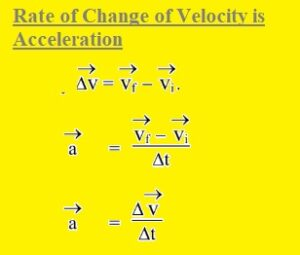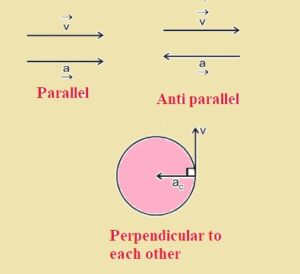Hello dear FSC students welcome to new tutorial. Her we will discuss 1st year physics chapter 3 solved exercise. In this post we will discuss chapter 3 short question of FSC physics boo that will be helpful for you in your coming exams. Here I have explained all short question given in exercise of book in simple way. So let get started.

## 1st year physics chapter 3 solved exercise

### Q 3.1 Solution

• Uniform Velocity : Velocity is uniform it covers equal displacement in equal time interval.
• Variable Velocity: Velocity of body is variable if it covers unequal displacement in unequal time duration or interval.
• Acceleration on basis of Variable Velocity
• According to variable velocity rate of change of velocity is known as acceleration.
• Let us suppose that an object is moving with velocity vi and after some time interval velocity changes Vi to Vf. SO change in velocity that is acceleration is mention here.• SI unit of velocity is meter per second, m/s
• SI unit of acceleration is meter per second square.

### Q 3.2 Solution

• When object thrown upward sign of acceleration is negative with respect to velocity due to gravity.
• While if object through down acceleration is positive since acceleration and velocity has same direction

### Q 3.3 Solution

• Yes velocity of body can be reverse if value of acceleration is constant
• If any object is through upward in vertical direction its velocity reduces, velocity direction is upward and acceleration direction is downward according to gravity. After reaching the object at highest point velocity is zero.
• If object move downward its velocity direction is downward and acceleration direction also downward due to gravity so direction of acceleration is constant and velocity direction is reverse.

### Q 3.4 Solution

• a: it is incorrect statement object can not have constant velocity if speed is changing.
• b: Yes it can happen if any object moving in circular path.
• c: Yes it can happen if body moving upward and reaches at highest point than have zero velocity but have acceleration value that is a=g
• d: yes it is correct object can have constant acceleration of velocity reverse.

### Q 3.5 Solution

• Both balls reaches with same speed on ground but with different time. The velocity of ball through upward is Vi when comes back has same velocity Vi and passes to man so values of initial velocities for both balls is same hence final velocities will also same.

### Q 3.6 Solution

• a: Parallel: When velocity is increasing about the straight path then velocity and acceleration are parallel.
• b: Parallel: if velocity is decreasing about the straight path then acceleration and velocity are anti parallel.
• c: Perpendicular to each other: If care moves in circular path then velocity and acceleration are perpendicular to each other
• d: V is zero a is not zero: If brakes applied velocity becomes zero and car in rest position since acceleration in opposite direction.
• d: a is zero v is not zero:  acceleration is zero if car moving with uniform velocity.### Q 3.7 Solution

• Yes it is correct motion with constant velocity is specials case with constant acceleration.
• If object moves with constant velocity then acceleration is zero. So no change in velocity acceleration has zero value that is constant and 0 is constant. So motion with constant velocity is specials case for constant acceleration.

### Q 3.8 Solution

• Let us suppose that body having mass m moving with velocity vi. If force F is applied on that objects its velocity changes vi to vf on the basis of first motion equation.
• Vf=vi+at
• a=(vf-vi)/t
• second law of motion says
• F=ma
• F=m(vf-vi)t
• F=(mvf-mvi)/t
• Here mvf is final momentum and mvi is initial momentum
• (mvf-mvi)/t is rate of change of momentum
• F is rate of changed momentum
• Newton second law of motion in form of momentum is rate of change of momentum is equal to applied force.

### Q 3.9 Solution

• Impulse: If large force applied on the objects for small time interval then product of this force and time is known as impulse. That is vector quantity
• Impulse = Force x Time
• I=F x Δt
• F x Δt=mvf-mvi
• as
• I=mvf-mvi
• I=m ΔV
• I= Δp that is change in momentum
• Hence impulse is equal to change in momentum

### Q 3.10 Solution

• Law of conservation of linear momentum
• According to this law total linear momentum of isolated system is constant.
• Isolated System Importance
• This law is applicable only for isolated system. System over which there is no external force applied called isolated system
• If any system is not isolated but external forces are small then the interacting forces then this law is applicable for this system.

### Q 3.11 Solution

• Elastic Collision:  Collision has constant value of K.E called elastic collision.
• Inelastic Collision: Collision that not have constant value of K.E is called inelastic collisions.
• For Bouncing Ball: If ideal bouncing come back to same height form it bounced it called elastic collision. If ball not come to same height than it is inelastic collision as energy changes that means K.E varies.
• Example: If heavy ball drop on the eart will not comes to dropped point since K.E lost and converted in the sound and heat energy. There for K.E is not constant in many situation.
• Momentum and K.E are conserved on each type of collision while for Eleatic collision only K.E is constant.

### Q 3.13 Solution

• Projectile motion has maximum speed at projection point and when it hit the ground. Its speed is minimum when reaches highest point.

That is all about the 1st year physics chapter 3 solved exercise. Dear students I have explained chapter 3 questions if you have any further query ask in comments. In coming post will discuss chapter 4 question.